# How to Filter Unique Records in Excel

In this article, we will learn How to Filter Unique Records in Excel.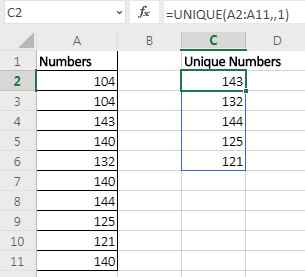Earlier in Excel 2016 and older versions we used complex formulas to extract unique values from a range. In Excel 365 online version, MS provides a new dynamic array function UNIQUE that simply returns the uniques from a given list.

Syntax of UNIQUE Function

 =UNIQUE(array,[by_col],[exactly_once])

Array: The array from which you want to extract unique values:

[by_col]: Set it TRUE(1) if the array is horizontal. By default it is FALSE for vertical data.

[exactly_once]: set it TRUE(1) if you want extract values that occur only once in the array. By default it is FALSE(0) to extract all unique values.

Let us have some examples to understand how this UNIQUE function works.

#### Example of UNIQUE Function

Example 1: Extract unique numbers from the list using UNIQUE function of Excel 365.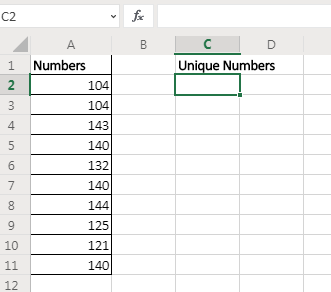Here I have sample data. In range A2:A11 I have some numbers that contain duplicate values. I want to get unique values from this range. I will simply use the UNIQUE function of Excel 365.

 =UNIQUE(A2:A11)

This formula simply returns all unique values from the range.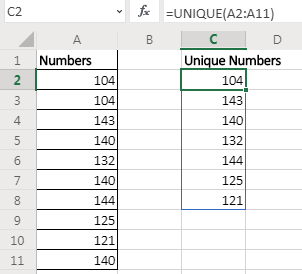As soon as you hit the enter button, the resultant array of unique value is spilled down the cells. This is a dynamic array that is only available for Excel 365 online.

Example 2: Extract Values that only occur once in the range.

In the above example, we got unique values from the range. If a value was occurring once, twice, or more we got only one stance of it. But if we want to extract values that only occurs once in the range (unique values in range) then the formula will be:

 =UNIQUE(A2:A11,1)Here we set the exactly_once variable to TRUE. This returns the value that are unique in the range/array itself.

Example 3: Find Unique Values in Horizontal Data

In the above two examples, the array was vertical and had numeric values. What if we have non numeric values and horizontal data? Well, not to worry. The excel UNIQUE function works on any kind of value be it numeric, text, boolean, etc. And we can use it on horizontal data setup too.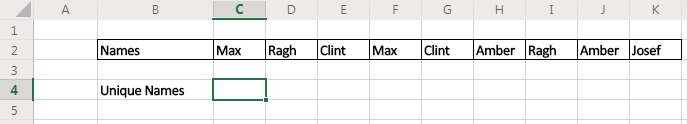Here I have some names in cells of adjacent columns. I want to get all names without repetition in the cells below.

The formula will be:

 =UNIQUE(C2:K2,,1)

It returns the names after removing all duplicates from the array in columns.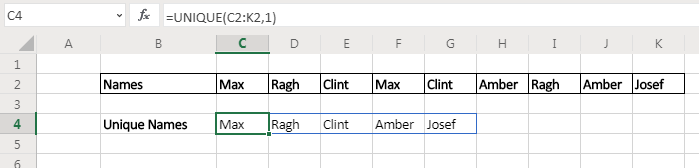For Excel 2010 - 2016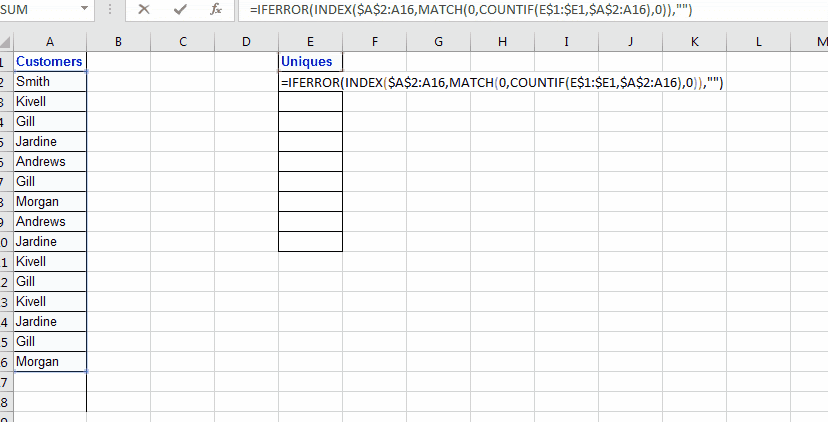To extract list of unique values from a list, we will use INDEX, MATCH and COUNTIF. I will also use IFERROR, just to have clean results, its optional.

And yes, it will be an array formula… So lets get it done…

Generic Formula Extract Unique Values in Excel

 {=INDEX(ref_list,MATCH(0,COUNTIF(expanding_ouput_range,ref_list),0))}

Ref_list: The list from which you want to extract unique values

Expanding_ouput_range: Now this is very important. This is the range where you want to see your extracted list. This range must have a distinct heading which is not a part in list and your formula will be below heading( if heading is in E1 than Formula will be in E2).

Now expanding means, when you drag down your formula it should expand over output range. To do so, you need to give reference of heading as \$E\$1:E1 (My heading is in E1). When I will drag it down, it will expand. In E2, it will be \$E\$1:E1. In E3, it will be \$E\$1:E2. In E2, it will be \$E\$1:E3 and so on.

Now lets see an example. It will make it clear.

#### Extracting Unique Values Excel Example

So here I have this list of customers in Column A in range A2:A16. Now in column E, I want to get unique values only from customers. Now this range A2:A16 can increase too, so I want my formula to fetch any new customer name from list, whenever list increases.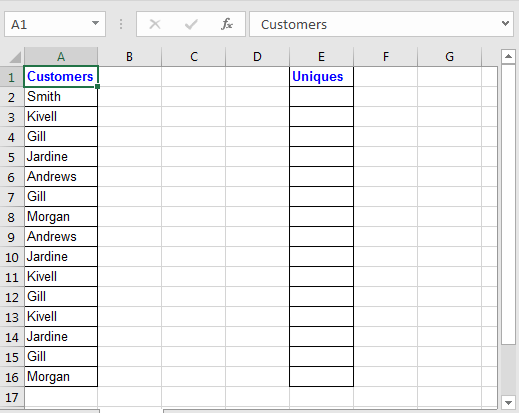Ok, Now to fetch unique values from Column A write this formula in Cell E2, and hit CTRL+SHIFT+ENTER to make it an array formula.

 {=INDEX(A\$2:A16,MATCH(0,COUNTIF(\$E\$1:E1,\$A\$2:A16),0))}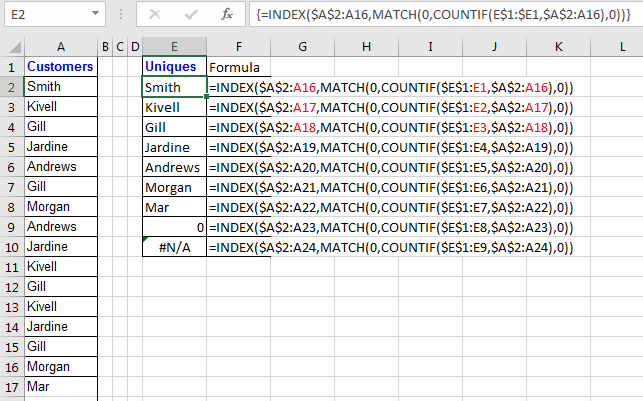A\$2:A16: I expect that list will expand and may have new unique values that I will like to extract. That is why I have left it open from bottom by not absolute reference of A16. It will allow it to expand whenever you copy formula bellow.

So we know how INDEX and MATCH function works. The main part here is:

COUNTIF(\$E\$1:E1,\$A\$2:A16): This formula will return an array of 1s and 0s. Whenever a value in range \$E\$1:E1 is found in criteria list \$A\$2:A16, the value convert into 1 at its position in range \$A\$2:A16.

Now using MATCH function we are looking for values 0. Match will return position of first 0 found in array returned by COUNTIF function. Than INDEX will look into A\$2:A16 to return value found at index returned by MATCH function.

It maybe a little bit hard to grasp but it works. The 0 in the end of the list indicates that there are no more unique values. If you don't see that 0 in the end you should copy the formula in below cells.

Now to avoid #NA in you can use IFERROR function of excel.

 {=IFERROR(INDEX(\$A\$2:A16,MATCH(0,COUNTIF(E\$1:\$E1,\$A\$2:A16),0)),"")}

Note: Don't put these curly braces manually, use Ctrl + Shift + Enter to get the result.

Hope this article about How to Filter Unique Records in Excel in Excel is explanatory. Find more articles on extracting values and related Excel formulas here. If you liked our blogs, share it with your friends on Facebook. And also you can follow us on Twitter and Facebook. We would love to hear from you, do let us know how we can improve, complement or innovate our work and make it better for you. Write to us at info@exceltip.com.

Related Articles :

Excel Formula to Extract Unique Values From a List | To find all unique values from a list in Excel 2016 and older versions, we use this simple formula.

UNIQUE Function for Excel 2016 | The Excel 2016 does not provide the UNIQUE function but we can create one. This UDF UNIQUE function returns all unique values from the list.

How To Count Unique Values in Excel With Criteria | To count unique values in excel with criteria we use a combination of functions. The FREQUENCY function is core of this formula

Popular Articles :

How to use the IF Function in Excel : The IF statement in Excel checks the condition and returns a specific value if the condition is TRUE or returns another specific value if FALSE.

How to use the VLOOKUP Function in Excel : This is one of the most used and popular functions of excel that is used to lookup value from different ranges and sheets.

How to Use SUMIF Function in Excel : This is another dashboard essential function. This helps you sum up values on specific conditions.

How to use the COUNTIF Function in Excel : Count values with conditions using this amazing function. You don't need to filter your data to count specific values. Countif function is essential to prepare your dashboard.

Terms and Conditions of use

The applications/code on this site are distributed as is and without warranties or liability. In no event shall the owner of the copyrights, or the authors of the applications/code be liable for any loss of profit, any problems or any damage resulting from the use or evaluation of the applications/code.